• Artificial Intelligence
• OCT 12, 2018

The Deep Learning Dictionary

Ready to learn Artificial Intelligence? Browse courses like  Uncertain Knowledge and Reasoning in Artificial Intelligence developed by industry thought leaders and Experfy in Harvard Innovation Lab.

Ever struggle to recall what Adam, ReLU or YOLO mean? Look no further and check out every term you need to master Deep Learning.

Surviving in the Deep Learning world means understanding and navigating through the jungle of technical terms. You’re not sure what AdaGrad, Dropout, or Xavier Initialization mean? Use this guide as a reference to freshen up your memory when you stumble upon a term that you safely parked in a dusty corner in the back of your mind.

This dictionary aims to briefly explain the most important terms of Deep Learning. It contains short explanations of the terms, accompanied by links to follow-up posts, images, and original papers. The post aims to be equally useful for Deep Learning beginners and practitioners.

Let’s open the encyclopedia of deep learning.Activation Function— Used to create a non-linear transformation of the input. The inputs are multiplied by weights and added to a bias term. Popular Activation functions include ReLU, tanh or sigmoid.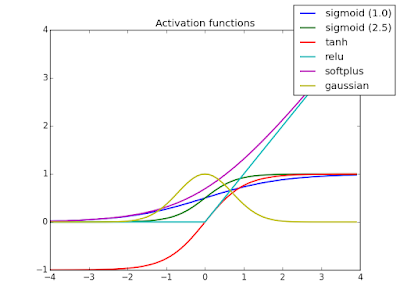Source: https://bit.ly/2GBeocg

Adam Optimization — Can be used instead of stochastic gradient descent optimization methods to iteratively adjust network weights. Adam is computationally efficient, works well with large data sets, and requires little hyperparameter tuning, according to the inventors. Adam uses an adaptive learning rate α, instead of a predefined and fixed learning rate. Adam is currently the default optimization algorithm in deep learning models.

Average Pooling — Averages the results of a convolutional operation. It is often used to shrink the size of an input. Average pooling was primarily used in older Convolutional Neural Networks architectures, while recent architectures favor maximum pooling.

AlexNet — A popular CNN architecture with eight layers. It is a more extensive network architecture than LeNet and takes longer to train. AlexNet won the 2012 ImageNet image classification challenge. Read the paper here.

Source: https://goo.gl/BVXbhL

Backpropagation —The general framework used to adjust network weights to minimize the loss function of a neural network. The algorithm travels backward through the network and adjusts the weights through a form of gradient descent of each activation function.

Backpropagation travels back through the network and adjusts the weights

Batch Gradient Descent — Regular gradient descent optimization algorithm. Performs parameter updates for the entire training set. The algorithm needs to calculate the gradients of the whole training set before completing a step of parameter updates. Thus, batch gradient can be very slow for large training sets.

Batch Normalization — Normalizes the values in a neural network layer to values between 0 and 1. This helps train the neural network faster.

Bias —Occurs when the model does not achieve a high accuracy on the training set. It is also called underfitting. When a model has a high bias, it will generally not yield high accuracy on the test set.

Source: https://goo.gl/htKsQS

Classification — When the target variable belongs to a distinct class, not a continuous variable. Image classification, fraud detection or natural language processing are examples of deep learning classification tasks.

Convolution — A mathematical operation which multiplies an input with a filter. Convolutions are the foundation of Convolutional Neural Networks, which excel at identifying edges and objects in images.

Cost Function — Defines the difference between the calculated output and what it should be. Cost functions are one of the key ingredients of learning in deep neural networks, as they form the basis for parameter updates. The network compares the outcome of its forward propagation with the ground-truth and adjusts the network weights accordingly to minimize the cost function. The root mean squared error is a simple example of a cost function.

Deep Neural Network — A neural network with many hidden layers, usually more than five. It is not defined how many layers minimum a deep neural network has to have. Deep Neural Networks are a powerful form of machine learning algorithms which are used to determine credit risk, steer self-driving cars and detect new planets in the universe.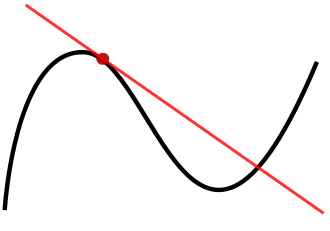Derivative of a function. Source: https://goo.gl/HqKdeg

Derivative — The derivative is the slope of a function at a specific point. Derivatives are calculated to let the gradient descent algorithm adjust weight parameters towards the local minimum.

Dropout — A regularization technique which randomly eliminates nodes and its connections in deep neural networks. Dropout reduces overfitting and enables faster training of deep neural networks. Each parameter update cycle, different nodes are dropped during training. This forces neighboring nodes to avoid relying on each other too much and figuring out the correct representation themselves. It also improves the performance of certain classification tasks. Read the paper here.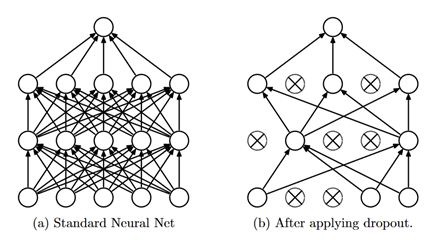Source: https://goo.gl/obY4L5

End-to-End Learning — An algorithm is able to solve the entire task by itself. Additional human intervention, like model switching or new data labeling, is not necessary. For example, end-to-end driving means that the neural network figures out how to adjust the steering command just by evaluating images.

Epoch —Encompasses a single forward and backward pass through the training set for every example. A single epoch touches every training example in an iteration.

Forward Propagation — A forward pass in deep neural networks. The input travels through the activation functions of the hidden layers until it produces a result at the end. Forward propagation is also used to predict the result of an input example after the weights have been properly trained.

Fully-Connected layer — A fully-connected layer transforms an input with its weights and passes the result to the following layer. This layer has access to all inputs or activations from the previous layer.

Gated Recurrent Unit —A Gated Recurrent Unit (GRU) conducts multiple transformations on the given input. It is mostly used in Natural Language Processing Tasks. GRUs prevent the vanishing gradients problem in RNNs, similar to LSTMs. In contrast to LSTMs, GRUs don’t use a memory unit and are thus more computationally efficient while achieving a similar performance. Read the paper here.

No forget gate, in contrast to LSTM. Source: https://goo.gl/dUPtdV

Human-Level Performance — The best possible performance of a group of human experts. Algorithms can exceed human-level performance. Valuable metric to compare and improve neural network against.

Hyperparameters — Determine performance of your neural network. Examples of hyperparameters are, e.g. learning rate, iterations of gradient descent, number of hidden layers, or the activation function. Not to be confused with parameters or weights, which the DNN learns itself.

ImageNet — Collection of thousands of images and their annotated classes. Very useful resource for image classification tasks.

Iteration — Total number of forward and backward passes of a neural network. Every batch counts as one pass. If your training set has 5 batches and trains 2 epochs, then it will run 10 iterations.

Gradient Descent — Helps Neural Network decide how to adjust parameters to minimize the cost function. Repeatedly adjust parameters until the global minimum is found. This post contains a well-explained, holistic overview of different gradient descent optimization methods.

Source: https://bit.ly/2JnOeLR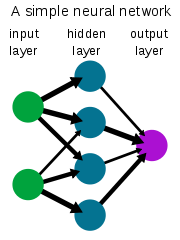Layer — A set of activation functions which transform the input. Neural networks use multiple hidden layers to create output. You generally distinguish between the input, hidden, and output layers.

Learning Rate Decay — A concept to adjust the learning rate during training. Allows for flexible learning rate adjustments. In deep learning, the learning rate typically decays the longer the network is trained.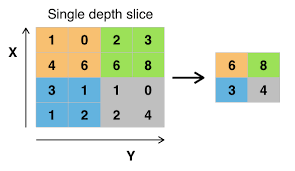Max pooling.

Maximum Pooling — Only selects the maximum values of a specific input area. It is often used in convolutional neural networks to reduce the size of the input.

Long Short-Term Memory — A special form of RNN which is able to learn the context of an input. While regular RNNs suffer from vanishing gradients when corresponding inputs are located far away from each other, LSTMs can learn these long-term dependencies. Read the paper here.

Input and Output of an LSTM unit. Source: https://bit.ly/2GlKyMF

Mini-Batch Gradient Descent— An optimization algorithm which runs gradient descent on smaller subsets of the training data. The method enables parallelization as different workers separately iterate through different mini-batches. For every mini-batch, compute the cost and update the weights of the mini-batch. It’s an efficient combination of batch and stochastic gradient descent.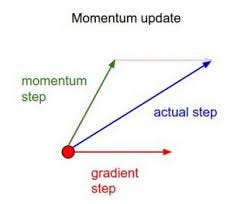Source: https://bit.ly/2Iz7uob

Momentum — A gradient descent optimization algorithm to smooth the oscillations of stochastic gradient descent methods. Momentum calculates the average direction of the direction of the previously taken steps and adjusts the parameter update in this direction. Imagine a ball rolling downhill and using this momentum when adjusting to roll left or right. The ball rolling downhill is an analogy to gradient descent finding the local minimum.

Neural Network — A machine learning model which transforms inputs. A vanilla neural network has an input, hidden, and output layer. Neural Networks have become the tool of choice for finding complex patterns in data.

Non-Max Suppression — Algorithm used as a part of YOLO. It helps detect the correct bounding box of an object by eliminating overlapping bounding boxes with a lower confidence of identifying the object. Read the paper here.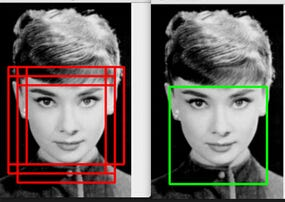Source:https://bit.ly/2H303sF

Recurrent Neural Networks — RNNs allow the neural network to understand the context in speech, text or music. The RNN allows information to loop through the network, thus persisting important features of the input between earlier and later layers.

Source: https://goo.gl/nr7Hf8

ReLU— A Rectified Linear Unit, is a simple linear transformation unit where the output is zero if the input is less than zero and the output is equal to the input otherwise. ReLU is the activation function of choice because it allows neural networks to train faster and it prevents information loss.

Regression —Form of statistical learning where the output variable is a continuous instead of a categorical value. While classification assigns a class to the input variable, regression assigns a value that has an infinite number of possible values, typically a number. Examples are the prediction of house prices or customer age.

Root Mean Squared Propagation — RMSProp is an extension of the stochastic gradient descent optimization method. The algorithm features a learning rate for every parameter, but not a learning rate for the entire training set. RMSProp adjusts the learning rate based on how quickly the parameters changed in previous iterations. Read the paper here.

Parameters — Weights of a DNN which transform the input before applying the activation function. Each layer has its own set of parameters. The parameters are adjusted through backpropagation to minimize the loss function.

Weights of a neural network

Softmax — An extension of the logistic regression function which calculates the probability of the input belonging to every one of the existing classes. Softmax is often used in the final layer of a DNN. The class with the highest probability is chosen as the predicted class. It is well-suited for classification tasks with more than two output classes.

Source: https://bit.ly/2HdWZHL

Stochastic Gradient Descent — An optimization algorithm which performs a parameter update for every single training example. The algorithm converges usually much faster than batch gradient descent, which performs a parameter update after calculating the gradients for the entire training set.

Supervised Learning — Form of Deep Learning where an output label exists for every input example. The labels are used to compare the output of a DNN to the ground-truth values and minimize the cost function. Other forms of Deep Learning tasks are semi-supervised training and unsupervised training.

Transfer Learning — A technique to use the parameters from one neural network for a different task without retraining the entire network. Use weights from a previously trained network and remove output layer. Replace the last layer with your own softmax or logistic layer and train network again. Works because lower layers often detect similar things like edges which are useful for other image classification tasks.

Unsupervised Learning — A form of machine learning where the output class is not known. GANs or Variational Auto Encoders are used in unsupervised Deep Learning tasks.

Validation Set — The validation set is used to find the optimal hyperparameters of a deep neural network. Generally, the DNN is trained with different combinations of hyperparameters are tested on the validation set. The best performing set of hyperparameters is then applied to make the final prediction on the test set. Pay attention to balancing the validation set. If lots of data is available, use as much as 99% for the training, 0.5% for the validation and 0.5% the test set.

Vanishing Gradients — The problem arises when training very deep neural networks. In backpropagation, weights are adjusted based on their gradient, or derivative. In deep neural networks, the gradients of the earlier layers can become so vanishingly small, that the weights are not updated at all. The ReLU activation function is suited to address this problem because it doesn’t squash the input as much as other functions. Read the paper here.

Variance —Occurs when the DNN overfits to the training data. The DNN fails to distinguish noise from pattern and models every variance in the training data. A model with high variance usually fails to accurately generalize to new data.

Vector — A combination of values that are passed as inputs into an activation layer of a DNN.

VGG-16 — A popular network architecture for CNNs. It simplifies the architecture of AlexNet and has a total of 16 layers. There are many pretrained VGG models which can be applied to novel use cases through transfer learning. Read the paper here.

Xavier Initialization — Xavier initialization assigns the start weights in the first hidden layer so that the input signals reach deep into the neural network. It scales the weights based on the number of neurons and outputs. This way, it prevents the signal from either becoming too small or too large later in the network.

YOLO — You Only Look Once, is an algorithm to identify objects in an image. Convolutions are used to determine the probability of an object being in a part of an image. Non-max suppression and anchor boxes are then used to correctly locate the objects. Read the paper here.

I hope this dictionary helped you get a clearer understanding of the terms used in the deep learning world. Keep this guide handy when taking the Coursera Deep Learning Specialization to quickly look up terms and concepts.The Harvard Innovation LabMatching Providers